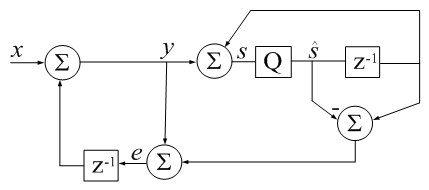# Accumulator Error Feedback

(Difference between revisions)
 Revision as of 20:17, 24 September 2017 (edit)← Previous diff Revision as of 18:20, 25 September 2017 (edit) (undo)Next diff → Line 17: Line 17: % See also SUM % See also SUM % % - % Matlab example: + % clear all - % v=rand(1e7,1); + % % v=sort(randn(13e6,1),'descend'); %better when sorted - % sum1 = sum(v); + % v=randn(13e6,1); - % sum2 = csum(v); + % rsumv = abs(sum(v) - sum(v(end:-1:1))); - % fprintf('sum1 = %18.16e\nsum2 = %18.16e\n', sum1, sum2); + % disp(['rsumv = ' num2str(rsumv,'%18.16f')]); + % csumv = abs(csum(v) - csum(v(end:-1:1))); + % disp(['csumv = ' num2str(csumv,'%18.16f')]); + % % vsumv = sum(vpa(v)) - sum(vpa(v(end:-1:1))); %vpa toolbox 32GB RAM + % % disp(['vsumv = ' char(vsumv)]) s_hat=0; e=0; s_hat=0; e=0; Line 33: Line 37: === links === === links === - [http://servidor.demec.ufpr.br/CFD/bibliografia/Higham_2002_Accuracy%20and%20Stability%20of%20Numerical%20Algorithms.pdf Accuracy and Stability of Numerical Algorithms, ch.4.3, Nicholas J. Higham, 1996] + [http://servidor.demec.ufpr.br/CFD/bibliografia/Higham_2002_Accuracy%20and%20Stability%20of%20Numerical%20Algorithms.pdf Accuracy and Stability of Numerical Algorithms 2e, ch.4.3, Nicholas J. Higham, 2002] For multiplier error feedback, see: For multiplier error feedback, see:

## Revision as of 18:20, 25 September 2017CSUM() in Digital Signal Processing terms: z-1 is a unit delay, Q is a floating-point quantizer to 64 bits, qi represents error due to quantization (additive by definition).
-Jon Dattorro
```function s_hat=csum(x)
% CSUM Sum of elements using a compensated summation algorithm.
%
% For large vectors, the native sum command in Matlab does
% not appear to use a compensated summation algorithm which
% can cause significant roundoff errors.
%
% This code implements a variant of Kahan's compensated
% summation algorithm which often takes about twice as long,
% but produces more accurate sums when the number of
% elements is large. -David Gleich
%
%
%  clear all
% % v=sort(randn(13e6,1),'descend');             %better when sorted
%  v=randn(13e6,1);
%  rsumv = abs(sum(v) - sum(v(end:-1:1)));
%  disp(['rsumv = ' num2str(rsumv,'%18.16f')]);
%  csumv = abs(csum(v) - csum(v(end:-1:1)));
%  disp(['csumv = ' num2str(csumv,'%18.16f')]);
% % vsumv = sum(vpa(v)) - sum(vpa(v(end:-1:1))); %vpa toolbox 32GB RAM
% % disp(['vsumv = ' char(vsumv)])

s_hat=0; e=0;
for i=1:numel(x)
s_hat_old = s_hat;
y = x(i) + e;
s_hat = s_hat_old + y;
e = (s_hat_old - s_hat) + y;  %calculate difference first (Higham)
end
```